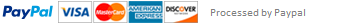### HS1031 Introduction to programming

Group Assignment Specifications

This assignment evaluates your understanding of basic programming principles using Python language. In particular, it assesses your ability to develop algorithms to solve simple problems, successfully develop and run python programs, and your ability to write meaningful comments when necessary. The assignment also provides a platform for students to work together in groups to develop solutions, which resembles how complex programming problems are solved in real-life.

1. Pythonize is a new language, that is similar to English, however the first letter of each word in Pythonize is moved to the end. Furthermore, each word in this new language ends with ‘py’. Write a program that takes an English word as an input and prints the Pythonize equivalent on the screen. (Save your script as pythonize.py and pythonize.txt)

For example:

If the input is: "Python" The output would be: Ythonppy

If the input is: "Programming" The output would be: Rogrammingppy

Notice that the first letter in a Pythonize word is capitalized (as it is the case in English).

2. Write a program that calculates the average of numeric values in a string and ignores all other characters. Your program must print the sum and average on the screen. (Save your script as spotNumbers.py and spotNumbers.txt)

For example:

If the input is: myString= "Biology = 66.0 Python = 90.0 Economics = 80.0 Statistics = 70.0"

The output wound be: Sum= 306.0 Average= 76.5

Hint: Consider using type build-in function in your code. Also, see documentation or exception handling in Python as it may prove to be handy in your code

3. Write a program that takes a string of words, and prints out these words one per line in a rectangular format (using # shape). The output must be properly formatted. (Save your script as tabularFormat.py and tabularFormat.txt)

For example:

If the input is: “Hello World! Python rules!” The output would be:

##########

# Hello    #

# World   #

# Python #

# rules    #

##########

This assignment has been answered 3 times in private sessions.